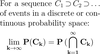Level 10 Level 12
Level 11

## Ignore words

Check the boxes below to ignore/unignore words, then click save at the bottom. Ignored words will never appear in any learning session.

Ignore?
Monotonicity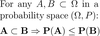Boole's inequality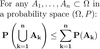Probability of complements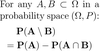Inclusion-exclusion principle (for probability of 2 events)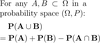Inclusion-exclusion principle (for probability of 3 events)Bayes' theorem (simple)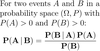Partition (of a set)Law of total probabilityBayes' theorem (extended)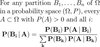Inclusion-exclusion principle (for probability of n events)(Monotone) Increasing sequence of events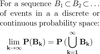(Monotone) Decreasing sequence of events